Limit of Sin(Φ) / Φ

The limit [ sin(Φ) / Φ ]  as Φ->0, is at first glance unknown as both the numerator and denominator

go to zero.   And division by zero is always undefined.  Nevertheless the result of the division does

approach a finite value. To derive this result, consider the following drawing.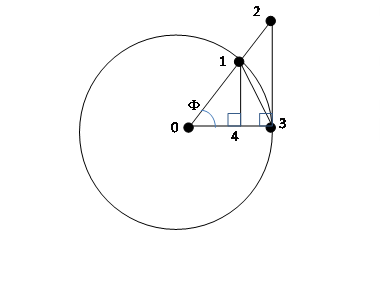The above circle has a radius of one so that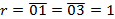The right triangle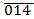has a height of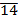= r sin(Φ) =  sin(Φ)

The right triangle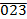has a height of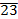= r tan(Φ) =  sin(Φ) / cos(Φ)

The area of that fraction of the circle in the “sector”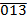(not the triangle) is

Asector = (Φ/(2π)) π r2 =  Φ/2

where the angle Φ is expressed in radians and the area includes that portion between the

circumference of the circle and the chord.

The area of the isosceles triangleis

A013 = (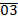) () / 2 = sin(Φ) / 2

The area of the right triangleis

A023 = () () / 2 = tan(Φ) / 2 = sin(Φ) / (2 cos(Φ))

Looking at the diagram above, the areas can be arranged in order as

A013  <  Asector  <  A023

and this can be rewritten as

sin(Φ) / 2  <  Φ/2  <  tan(Φ) / 2

or

1  <  Φ / sin(Φ)  <  1 / cos(Φ)

or finally by inverting each term and thus reversing the order

cos(Φ)  >  sin(Φ) / Φ  >  1

And because in the “limit” as the angle Φ gets smaller and smaller, we can write

limit(Φ->0) [ cos(Φ) ] = 1

then the term in the middle is “squeezed” to have the same value as at both ends or

limit(Φ->0) [ sin(Φ)  /  f ] = 1

which is what we finally wanted to show, namely that the ratio approaches a finite value in the limit.

And this is an important result especially for the derivatives of trigonometric functions.